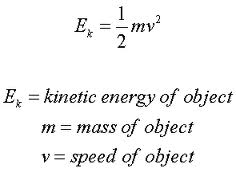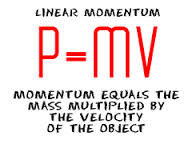# Difference between Kinetic Energy and Momentum

##### Key Difference: Kinetic energy is an energy that is possessed by an object, due to its motion. It is equivalent to the work that is required in order to accelerate the object. Momentum is described as a quantity that states an object’s resistance to stopping. Therefore, it can also be defined as the “Inertia in Motion.”Kinetic energy is an energy that is possessed by an object, due to its motion. It is equivalent to the work that is required in order to accelerate an object. Thus, we can also say that every moving object has kinetic energy. It is calculated by finding one half the mass of the object times square of its speed.Momentum is described as a quantity that states an object’s resistance to stopping. Therefore, it can also be defined as the “Inertia in Motion.” Momentum is possessed by any type of entity that is moving. Linear momentum is a product of mass and velocity. It is hard to stop a heavy vehicle in comparison to a light vehicle. This is due to the fact that the momentum of a heavy car is greater than a light vehicle, because of the car's greater mass. Impulse and momentum are vector quantities as both are products of a scalar and a vector quantity. Newton’s second law of motion is expressed as - the rate of change of momentum is directly proportional to the net force and is in the direction of that force.

Comparison between Impulse and Momentum:

 Kinetic Energy Momentum Definition Kinetic energy is an energy that is possessed by an object, due to its motion. It is equivalent to the work that is required in order to accelerate the object. Momentum is described as a quantity that states an object’s resistance to stopping. Impulse and momentum are directly related to each other. Formula Product of an object’s mass and square of velocity. K = 0.5 m x v². Product of an object’s mass and velocity. p = m x v. Type of force Force integrated over distance Force integrated over time Type Scalar Vector S.I. Unit Joules kg m/s Kilogram meter per second Conserved Conserved for an isolated system only as part of total energy budget. Conserved absolutely for an isolated system. Laws of conservation If a system experiences a net decrease (increase) in total kinetic energy, then the difference must be accounted for by an equal increase (decrease) in other forms of energy (e.g., heat, chemical potential, gravitational potential, electrostatic potential. etc.). Momentum of a system is constant if there are no external forces acting on the system. It is embodied in Newton's first law (the law of inertia).

Image Courtesy: astronomyonline.org, physics4kids.com